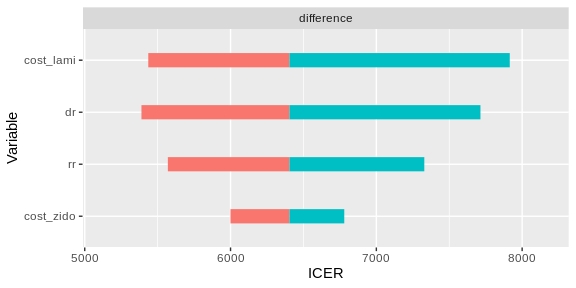Deterministic Sensitivity Analysis

Introduction

The objective of deterministic sensitivity analysis is to assess how model results are sensitive to parameter values. Parameter values are changed through upper and lower bounds, and the results are reported.

Sensitivity analysis is distinct from probabilistic uncertainty analysis: whereas in PSA the objective is to estimate the effect of global uncertainty on model results, in DSA the objective is to assess the sensitivity of results to variations of individual parameters. Both analyses are complementary.

Define the analysis

This example uses the HIV drug model defined in vignette("e-probabilistic", "heemod"). See this vignette for an explanation of the model. Note that as in PSA, parameters need to be defined in define_parameters() in order to be modified in a DSA.

In this example we will study the sensitivity of cost to 4 parameters:

• rr, the relative risk associated with the new treatment.
• cost_zido and cost_lami, the drug costs.
• dr, the discount rate.

Upper and lower values for the paramters are given to define_dsa().

se <- define_dsa(
rr, .4, .6,

cost_zido, 1500, 3000,
cost_lami, 1500, 3000,

dr, .04, .08
)

We then run the sensitivity analysis with run_dsa(), using res_mod the result from run_model() as input.

res_dsa <- run_dsa(
model = res_mod,
dsa = se
)
## Running DSA on strategy 'mono'...
## Running DSA on strategy 'comb'...

Interpretation

All the results can be displayed in a table.

res_dsa
## A sensitivity analysis on 4 parameters.
##
## Parameters:
##   -rr
##   -cost_zido
##   -cost_lami
##   -dr
##
## Sensitivity analysis:
##
##                        cost_health cost_drugs cost_total life_year
## mono, cost_lami = 1500 46725886    19279596   48417031    8463.387
## comb, cost_lami = 1500 71019861    53642502   79593035   14198.651
## mono, cost_lami = 3000 46725886    19279596   48417031    8463.387
## comb, cost_lami = 3000 71019861    74940478   93812382   14198.651
## mono, cost_zido = 1500 46725886    12695081   43363208    8463.387
## comb, cost_zido = 1500 71019861    50916361   77772959   14198.651
## mono, cost_zido = 3000 46725886    25390162   53107083    8463.387
## comb, cost_zido = 3000 71019861    72214337   91992306   14198.651
## mono, dr = 0.04        46725886    19279596   53238469    8463.387
## comb, dr = 0.04        71019861    61962911   97480846   14198.651
## mono, dr = 0.08        46725886    19279596   44351921    8463.387
## comb, dr = 0.08        71019861    61962911   75259528   14198.651
## mono, rr = 0.4         46725886    19279596   48417031    8463.387
## comb, rr = 0.4         74749377    69178956   89573275   15852.190
## mono, rr = 0.6         46725886    19279596   48417031    8463.387
## comb, rr = 0.6         66652719    56216918   80800674   12881.970
##                        .n_indiv .par_value_eval Cost     Effect   ICER
## mono, cost_lami = 1500 1000        0.40             0.00 0.000000 -
## comb, cost_lami = 1500 1000     1500.00         31176.00 5.735263 5435.845
## mono, cost_lami = 3000 1000        0.60             0.00 0.000000 -
## comb, cost_lami = 3000 1000     3000.00         45395.35 5.735263 7915.129
## mono, cost_zido = 1500 1000     1500.00             0.00 0.000000 -
## comb, cost_zido = 1500 1000        0.04         34409.75 5.735263 5999.681
## mono, cost_zido = 3000 1000     3000.00             0.00 0.000000 -
## comb, cost_zido = 3000 1000        0.08         38885.22 5.735263 6780.024
## mono, dr = 0.04        1000     1500.00             0.00 0.000000 -
## comb, dr = 0.04        1000        0.04         44242.38 5.735263 7714.097
## mono, dr = 0.08        1000     3000.00             0.00 0.000000 -
## comb, dr = 0.08        1000        0.08         30907.61 5.735263 5389.047
## mono, rr = 0.4         1000        0.40             0.00 0.000000 -
## comb, rr = 0.4         1000     1500.00         41156.24 7.388802 5570.083
## mono, rr = 0.6         1000        0.60             0.00 0.000000 -
## comb, rr = 0.6         1000     3000.00         32383.64 4.418583 7328.966
##                        Cost Diff. Effect Diff. Ref. .nmb     .dnmb
## mono, cost_lami = 1500 -          -            -         0.0 -
## comb, cost_lami = 1500 31176.00   5.735263     mono 140881.9 140881.9
## mono, cost_lami = 3000 -          -            -         0.0 -
## comb, cost_lami = 3000 45395.35   5.735263     mono 126662.5 126662.5
## mono, cost_zido = 1500 -          -            -         0.0 -
## comb, cost_zido = 1500 34409.75   5.735263     mono 137648.1 137648.1
## mono, cost_zido = 3000 -          -            -         0.0 -
## comb, cost_zido = 3000 38885.22   5.735263     mono 133172.7 133172.7
## mono, dr = 0.04        -          -            -         0.0 -
## comb, dr = 0.04        44242.38   5.735263     mono 127815.5 127815.5
## mono, dr = 0.08        -          -            -         0.0 -
## comb, dr = 0.08        30907.61   5.735263     mono 141150.3 141150.3
## mono, rr = 0.4         -          -            -         0.0 -
## comb, rr = 0.4         41156.24   7.388802     mono 180507.8 180507.8
## mono, rr = 0.6         -          -            -         0.0 -
## comb, rr = 0.6         32383.64   4.418583     mono 100173.8 100173.8

Two distinct plot types are available. The basic plot (type = "simple") displays cost variations for each model, around the base cost.

As expected mono model costs are not senstive to cost_lami, since this drug was not given to this group. Similarly it is not sensitive to rr, because this parameters only modifies transition probabilities in the other model.

plot(res_dsa,
strategy = "mono",
result = "cost",
type = "simple")
## Joining, by = ".strategy_names"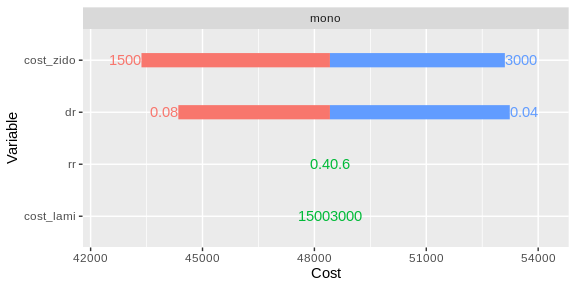On the other hand the comb model cost is sensitive to all 4 parameters.

plot(res_dsa,
strategy = "comb",
result = "cost",
type = "simple")
## Joining, by = ".strategy_names"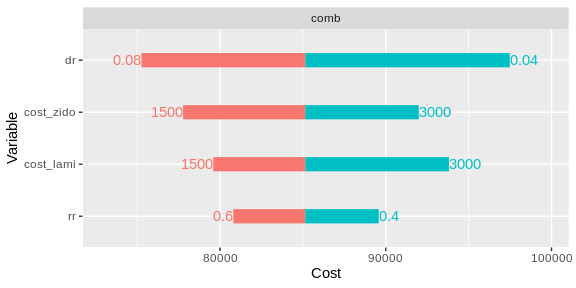And its effectiveness is sensitive to rr

plot(res_dsa,
strategy = "comb",
result = "effect",
type = "simple")
## Joining, by = ".strategy_names"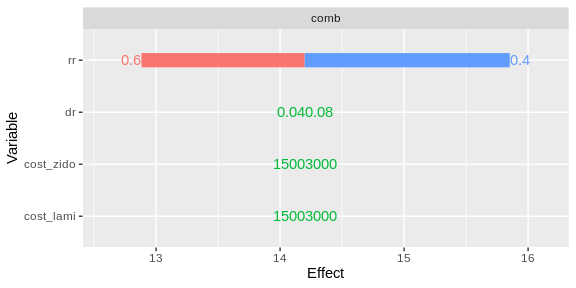The difference plot (type = "difference") displays the difference between the specified model comb and the reference model mono.

plot(res_dsa,
strategy = "comb",
result = "cost",
type = "difference")
## Joining, by = ".strategy_names"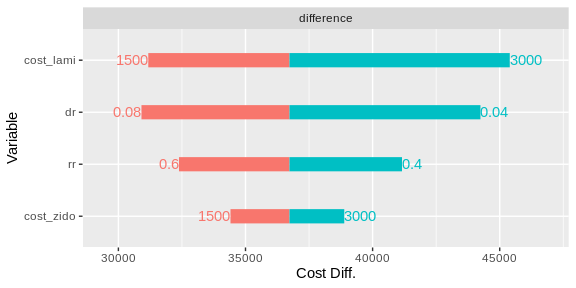plot(res_dsa,
strategy = "comb",
result = "icer",
type = "difference")
## Joining, by = ".strategy_names"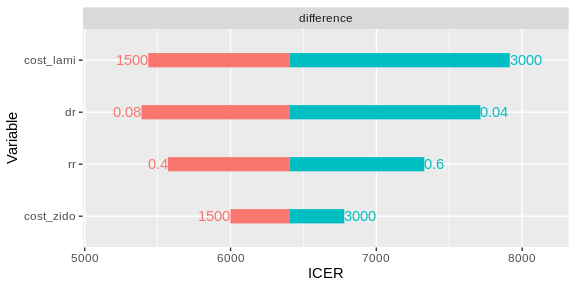It is also possible to leave the high and low parameter values off the plot:

plot(res_dsa,
strategy = "comb",
result = "icer",
type = "difference",
limits_by_bars = FALSE)
## Joining, by = ".strategy_names"